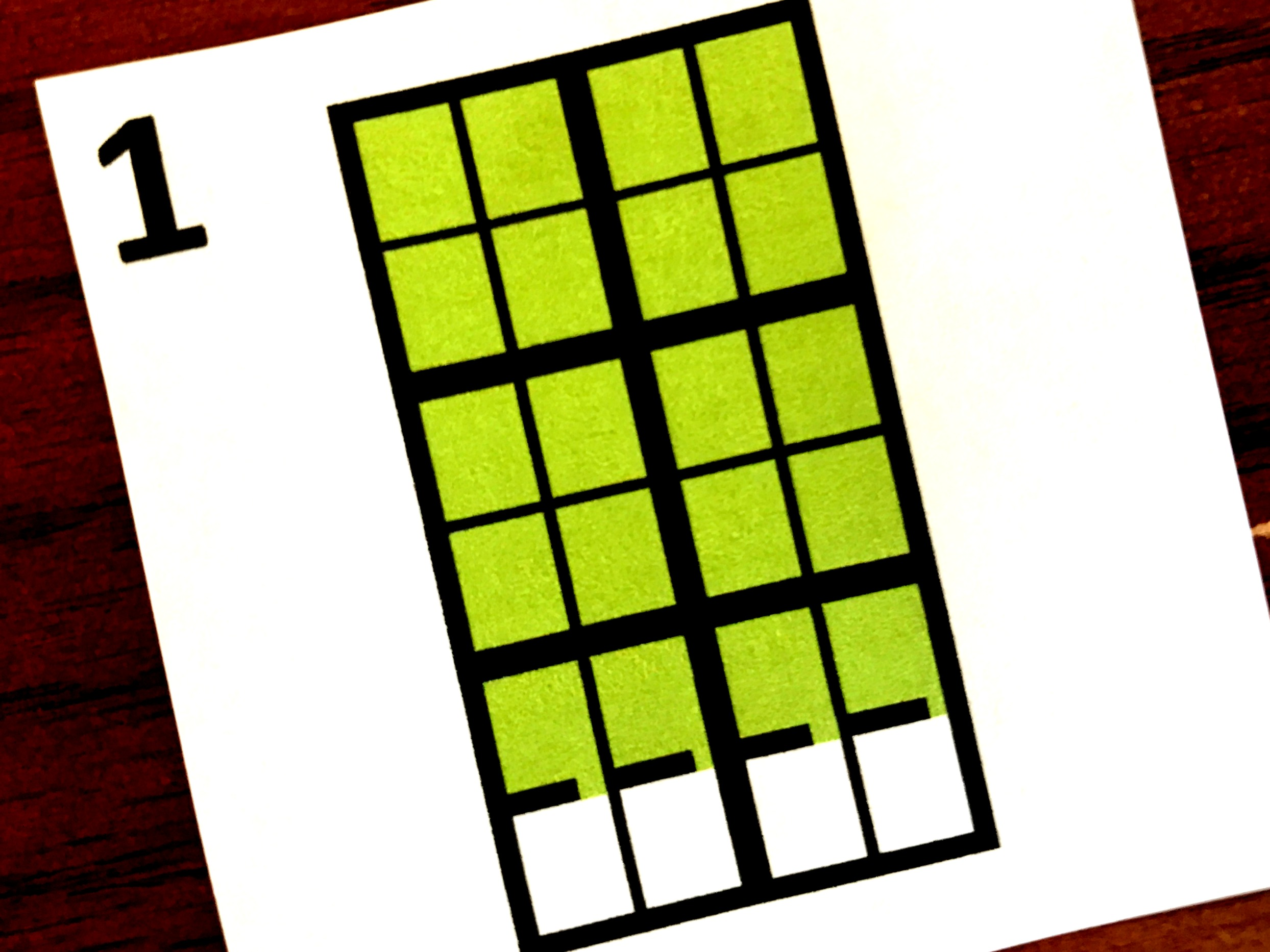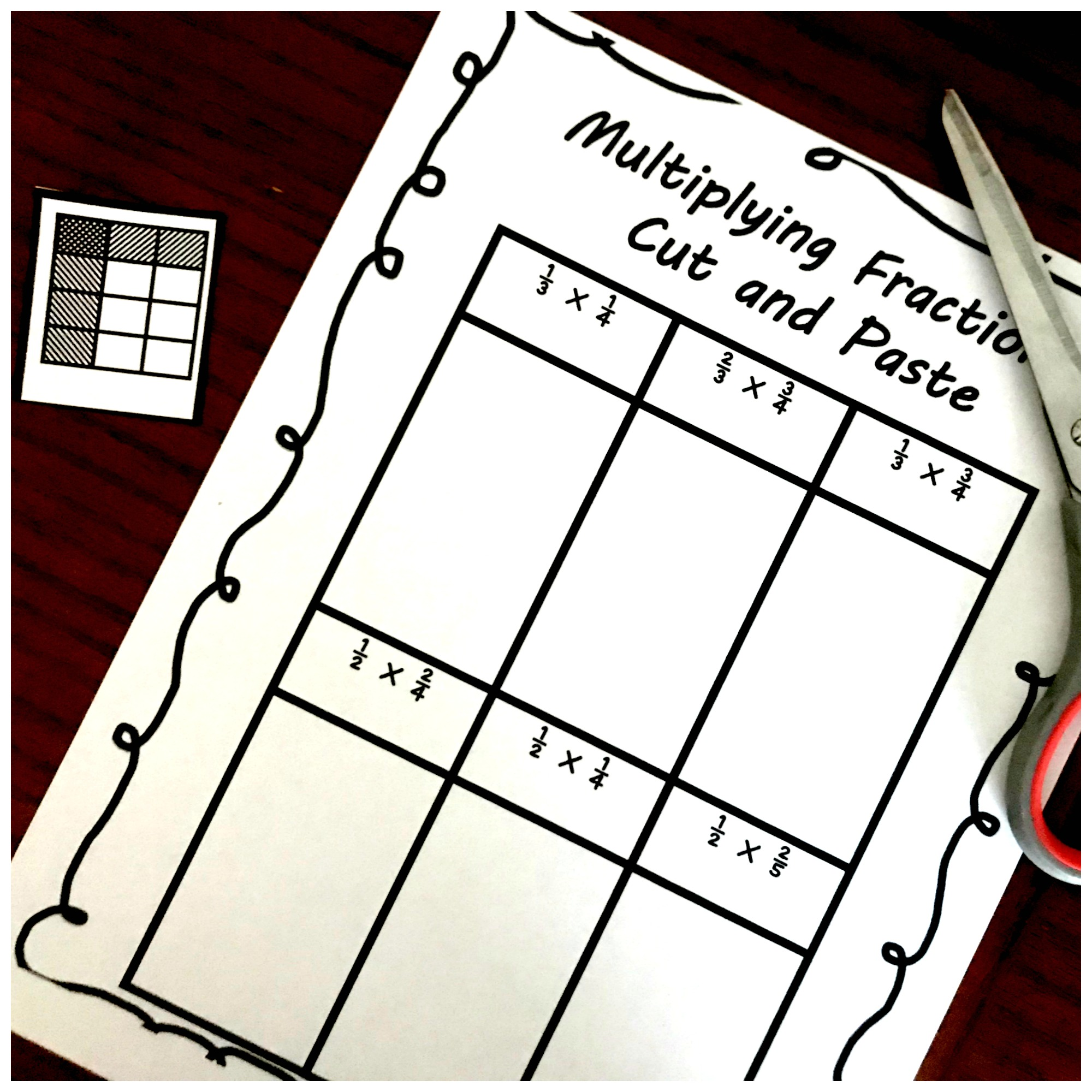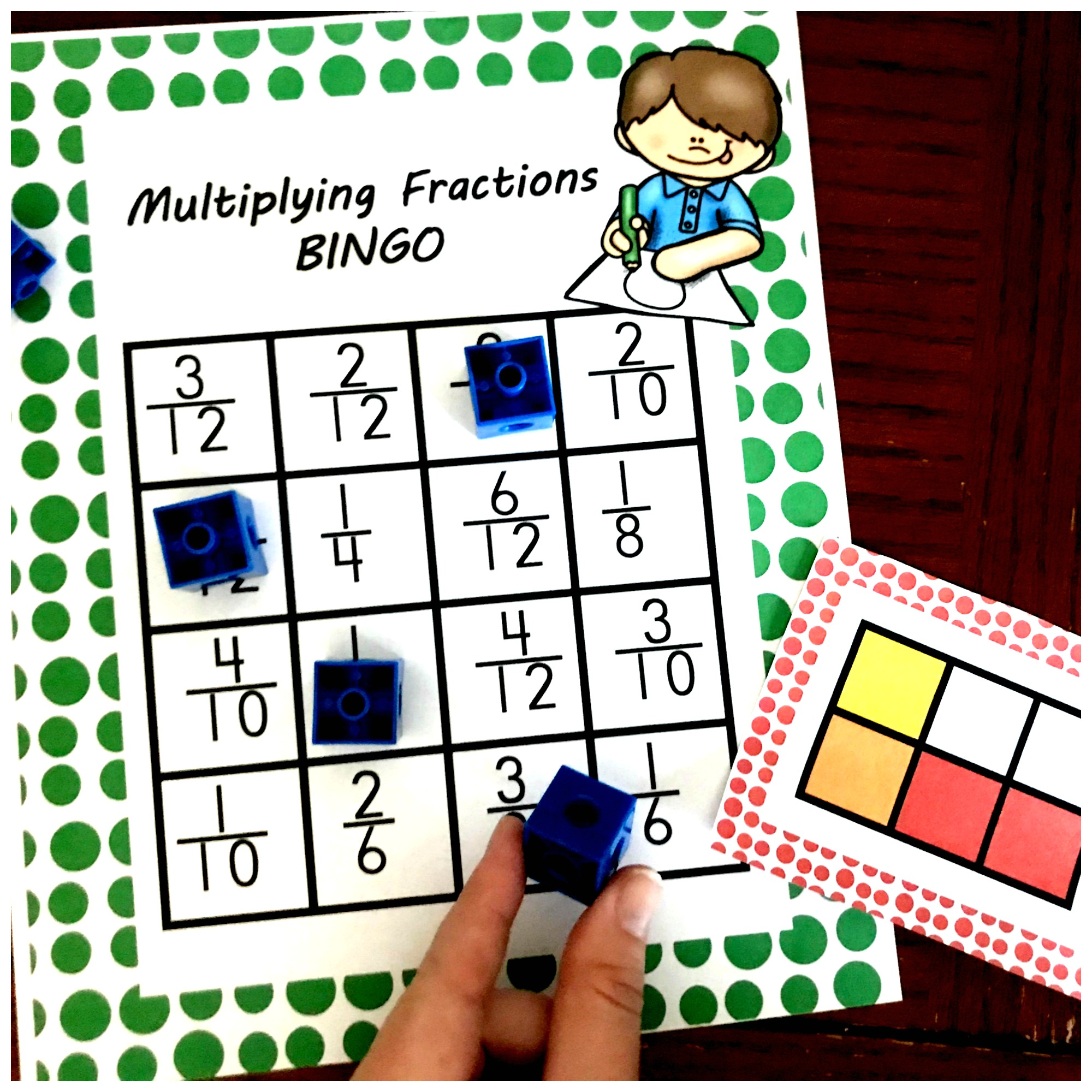Home » Math » 3 Cut and Paste Worksheets For Multiplying Fractions Practice

3 Cut and Paste Worksheets For Multiplying Fractions Practice

These three cut and paste worksheets are perfect for multiplying fractions practice. It is a fun way to use area models to solve fraction by fraction problems.We get to continue our focus on multiplying fractions by fractions today!! We have already had so much fun as we have learned to multiply fractions by fractions with an interactive notebook, and then moved onto a fun game. Today though, after the fun is a chance to show off what our kiddos know. And there is a little fun added to it since it is a cut and paste activity.

If you need fractions by whole number practice, this may help.Or multiplying mixed number practice is HERE.Prep Work –

Prep work for this activity couldn’t be simpler!!

• First, print off cut and paste sheets. Each child will need the page that has the expressions on it. The pages with the models need to be cut in half. Every child should receive a half!!
• Finally, gather up glue and scissors are you are ready to go!

Directions –

Now it is time for some multiplying fractions practice!

• First, the children need to cut out each of the models.• Next, it is time to figure out where each area model goes! In this example, you can see that the expression is 1/2 x 2/4. The array that is being placed down has been divided in half vertical. You can see that 1/2 of the shape has been colored in. Then if you look at the horizontal lines, you will see that the shape has been divided into fourths. Two of those fourths have been shaded in! This array equals 1/2 x 2/4.• Finally, the students write an answer underneath the array. The model has two parts shaded in darkly, so two is the numerator. The array has eight parts making eight the denominator. So the answer is 2/8. If you have your children simplify the answer, the answer is 1/4.

Hope this helps you get in some extra multiplying fractions practice with your children!!

You’ve Got This

Rachel

You may also like are multiplying fractions game for more practice:Or get all my multiplying fractions activities in one place: